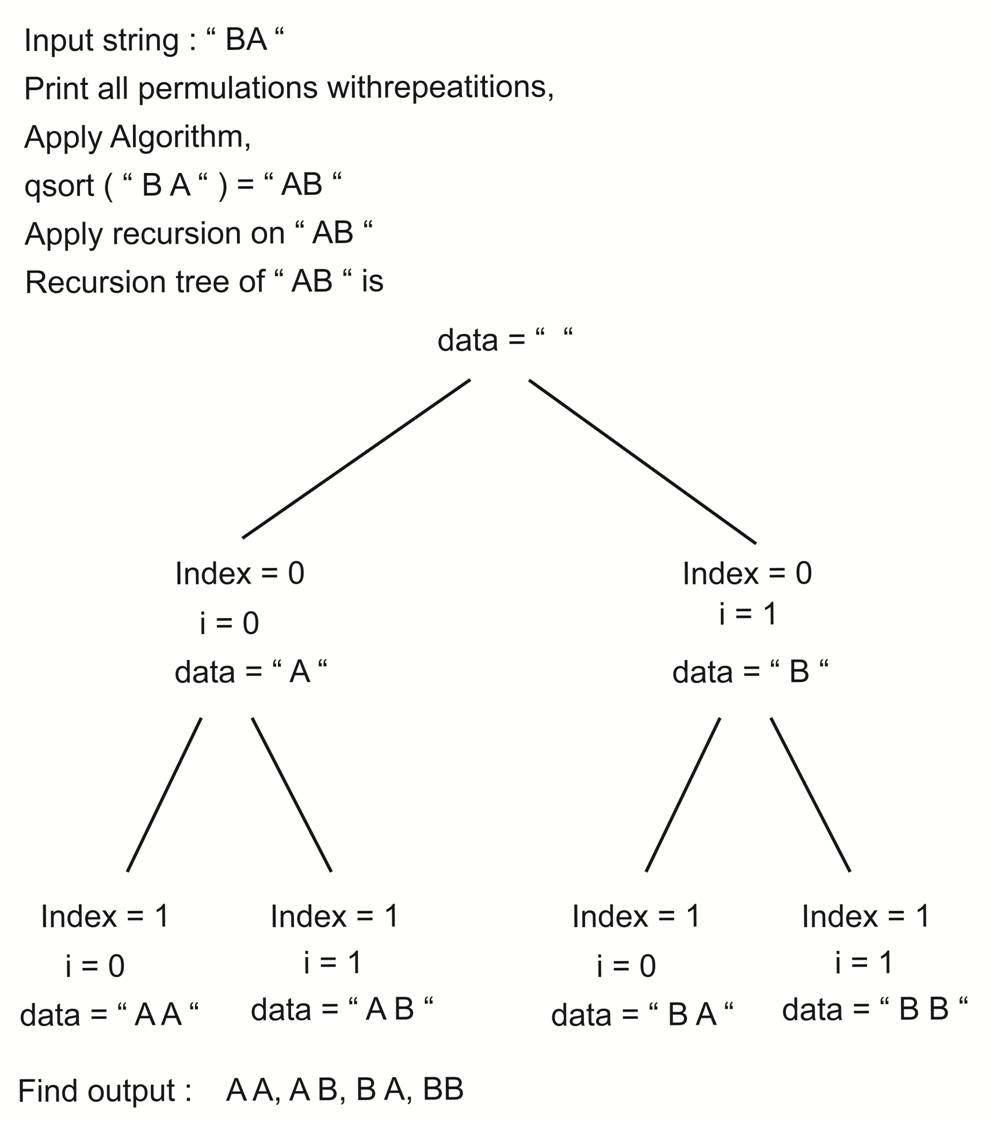# Print all permutations with repetition

## For the given input string, print all the possible permutations. Repetition of characters is allowed.

We should print them in lexicographic order.

### Example

a. Input string is: AB

Output:
AA
AB
BA
BB
Total 4 permutations.

b. Input string is: ABC

Output:
AAA, AAB, AAC, ABA, ABB, ABC, ACA, ACB, ACC,
BAA, BAB, BAC, BBA, BBB, BBC, BCA, BCB, BCC,
CAA, CAB, CAC, CBA, CBB, CBC, CCA, CCB, CCC
Total 27 permutations.

## Algorithm

Total number of possible permutations is nn

Here we use recursion,

a. We fix all characters one by one at the index and we use recursion for the subsequent indices.

b. We fix the ith character at index and if it is not the last index, then recursively call for higher indexes.

c. If it is the last index, we print the sting stored.

d. We need to do this lexicographic order, so we use inbuild function qsort to sort the characters of string and apply recursion on the sorted string.

### Algorithm working## C++ Program

```#include <bits/stdc++.h>

using namespace std;

int Compare(const void * a, const void * b)
{
return( *(char *)a - *(char *)b );
}
//Recursive function
//Not lexicographic order
void PrintPermutationsRecursion (char *str, char* data, int last, int index)
{
int i, length = strlen(str);

for ( i=0; i<length; i++ )
{
data[index] = str[i] ;
//print string if it is last index
if (index == last)
{
cout<<data<<endl;
}
//Recurstion for next index if not last index
else
{
PrintPermutationsRecursion(str, data, last, index+1);
}
}
}

//Print in lexicographic order
void PrintLexicographicOrder(char *str)
{
int length = strlen(str) ;
char *data = (char *) malloc (sizeof(char) * (length + 1)) ;
//Null termination
data[length] = '\0';
//sort input string in lexicographic order
qsort(str, length, sizeof(char), Compare);
//Call recursive function on sorted string
PrintPermutationsRecursion (str, data, length-1, 0);
//Free memory
free(data);
}

//Main function
int main()
{
char str[] = "CAB";
cout<<"Permutations with repetation for string "<<str<<" are: "<<endl;
PrintLexicographicOrder(str);
return 0;
}```

Translate »